16 Sep

## Dummy Classifier

IIt is useful when developing machine learning models, to be modeling against a benchmark. If your new model cannot beat your benchmark model, then we are just wasting time and could be doing something more productive somewhere else. There may be a time where you do not have access to the previous model and don't have an obvious model for benchmarking. In this case, we can use a Dummy Model, which follows a heuristic on the input data. The dummy model would always "classify records as being from group 1", or "classify records at random from the target space/distribution," outputting our performance metrics afterward. This notebook will review the outputs that you can expect from a dummy classifier. The model can be useful when examining the results of your model and communicating that information to your team.

Dataset

Poisonous Mushrooms Dataset: https://www.kaggle.com/uciml/mushroom-classification

### Import Preliminaries¶

In :
%matplotlib inline
%config InlineBackend.figure_format='retina'

# Import modules
import itertools
import matplotlib.pyplot as plt
import matplotlib as mpl
import numpy as np
import pandas as pd
import seaborn as sns
import warnings

from matplotlib import rcParams, gridspec
from sqlalchemy import create_engine
from sklearn.metrics import confusion_matrix
from sklearn.model_selection import train_test_split, KFold, cross_val_score
from sklearn.dummy import DummyClassifier
from sklearn.linear_model import LogisticRegression

# Set pandas options
pd.set_option('max_columns',1000)
pd.set_option('max_rows',30)
pd.set_option('display.float_format', lambda x: '%.3f' % x)

# Set plotting option
mpl.rcParams['figure.figsize'] = (9.0, 3.0)

# Set warning options
warnings.filterwarnings('ignore');

# Plot a confusion matrix function
def plot_confusion_matrix(cm, classes, fontsize=20,
normalize=False, title='Confusion matrix',
cmap=plt.cm.Blues):
'''
THE MAIN CONFUSION MATRIX, KAVI DON'T DELTETE BY ACCIDENT AGAIN. Function plots a
confusion matrix given a cm matrix and class names

Parameters
----------
cm: sklearn confusion matrix
classes: numpy 1D array containing all unique class names

Examples
---------
>>>>

plot_confusion_matrix(
cm,
classes,
fontsize=25,
normalize=True,
title=model.name.capitalize() + ': Test Set',
cmap=plt.cm.Greens)

'''
cm_num = cm
cm_per = cm.astype('float') / cm.sum(axis=1)[:, np.newaxis]

if normalize:
cm = cm.astype('float') / cm.sum(axis=1)[:, np.newaxis]
# print("Normalized confusion matrix")
else:
None
# print('Confusion matrix, without normalization')

# print(cm)

plt.imshow(cm, interpolation='nearest', cmap=cmap)
plt.title(title.replace('_',' ').title()+'\n', size=fontsize)
plt.colorbar()
tick_marks = np.arange(len(classes))
plt.xticks(tick_marks, classes, rotation=45, size=fontsize)
plt.yticks(tick_marks, classes, size=fontsize)

fmt = '.5f' if normalize else 'd'
thresh = cm.mean()
for i, j in itertools.product(range(cm.shape), range(cm.shape)):
# Set color parameters
color = "white" if cm[i, j] > thresh else "black"
alignment = "center"

# Plot perentage
text = format(cm_per[i, j], '.5f')
text = text + '%'
plt.text(j, i,
text,
fontsize=fontsize,
verticalalignment='baseline',
horizontalalignment='center',
color=color)
# Plot numeric
text = format(cm_num[i, j], 'd')
text = '\n \n' + text
plt.text(j, i,
text,
fontsize=fontsize,
verticalalignment='center',
horizontalalignment='center',
color=color)

plt.tight_layout()
plt.ylabel('True label'.title(), size=fontsize)
plt.xlabel('Predicted label'.title(), size=fontsize)

return None

# Plot a train and test confusion matrix together
def plot_confusion_normal(model, name, classes, train_x, train_y,
test_x, test_y, cmap=plt.cm.Greens):
'''
Fuction plota grid and calls the plot_confusion_matrix function
to plot two confusion matrices. One for the tarin set and another
for the test set

Parameters
----------
cm: sklearn confusion matrix
classes: numpy 1D array containing all unique class names

Examples
----------
>>>> plot_confusion_normal(xg_model, train_x, train_y)
>>>> plot_confusion_normal(rf_model, train_x, train_y)
'''

# Set the plot size
rcParams['figure.figsize'] = (15.0, 10.0)

# Set up grid
plt.figure()
fig = gridspec.GridSpec(3, 3)
grid_length = list(range(1, 3))
tuple_grid = [(i, j) for i in grid_length for j in grid_length]

# Plot Training Confusion Matrix
plt.subplot2grid((3, 3), (0, 0))
cm = confusion_matrix(train_y, model.predict(train_x))
plot_confusion_matrix(
cm,
classes,
fontsize=10,
normalize=True,
title=name.capitalize() + ': Train Set',
cmap=cmap)

# Plot Testing Confusion Matrix
plt.subplot2grid((3, 3), (0, 1))
cm = confusion_matrix(test_y, model.predict(test_x))
plot_confusion_matrix(
cm,
classes,
fontsize=10,
normalize=True,
title=name.capitalize() + ': Test Set',
cmap=cmap)

return None


### Import Data¶

In :
 # Set your local database parameters
host = '127.0.0.1'
port = '3306'
db_name = 'mushroom'

# Create a MySQLEngine
'+host+':'+port+'/'+db_name)

# Connect to database
engine.connect();

# Import data from SQL
df = pd.read_sql(""" SELECT * FROM mushrooms""", engine).dropna(how='any', axis=0)
df.rename(columns={'class':'target'}, inplace=True)
# Some preprocessing on the data

Out:
target cap-shape cap-surface cap-color bruises odor gill-attachment gill-spacing gill-size gill-color stalk-shape stalk-root stalk-surface-above-ring stalk-surface-below-ring stalk-color-above-ring stalk-color-below-ring veil-type veil-color ring-number ring-type spore-print-color population habitat
0 p x s n t p f c n k e e s s w w p w o p k s u
1 e x s y t a f c b k e c s s w w p w o p n n g
2 e b s w t l f c b n e c s s w w p w o p n n m
3 p x y w t p f c n n e e s s w w p w o p k s u
4 e x s g f n f w b k t e s s w w p w o e n a g
5 e x y y t a f c b n e c s s w w p w o p k n g
6 e b s w t a f c b g e c s s w w p w o p k n m
7 e b y w t l f c b n e c s s w w p w o p n s m
8 p x y w t p f c n p e e s s w w p w o p k v g
9 e b s y t a f c b g e c s s w w p w o p k s m

### Preprocess Data¶

In :
# One-Hot Encoding Data, and Label Encoding the target values
targets = df.target.astype('category').cat.codes
targets = pd.Series(targets, name='target')
encoded_df = pd.get_dummies(df.drop('target', axis=1))
encoded_df = pd.concat([encoded_df, targets ], axis=1)

X = encoded_df.drop('target',axis=1).values
y = encoded_df.target.values

# Conduct a train-test-split on the data
train_x, test_x, train_y, test_y = train_test_split(X,y, test_size=0.25)


### Data Overview¶

In :
# Plot a barplot of the target clasees in our training set (1 = posinous, 0 = eatable by humans)
pd.Series(train_y).value_counts().plot.barh(grid=False, color=['#CFCCC7','#6A6A6A','#000000'], width=0.25,edgecolor='w')
plt.title('Target Outcomes')
plt.ylabel('Target Class')
plt.xlabel('Number of Records');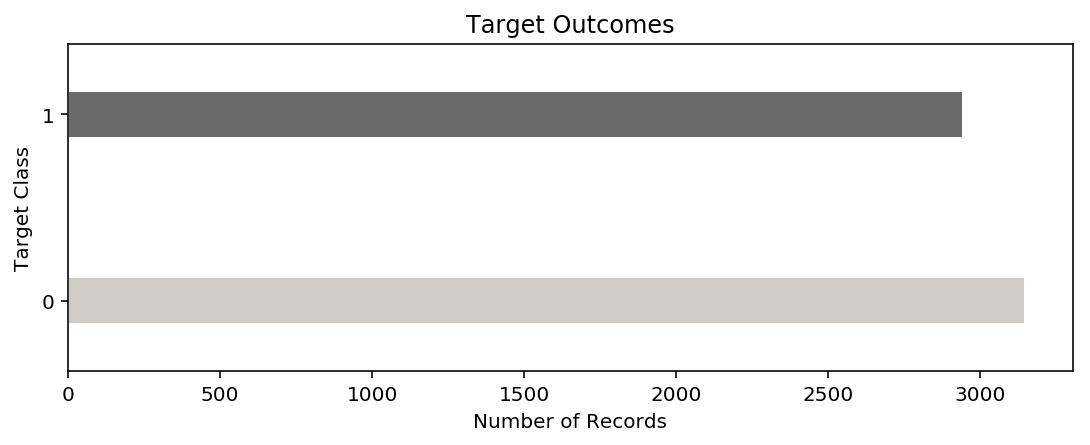### Constant Dummy Model¶

A constant model takes a constant value ("1") and tells us that everything is poisonous! The confusion matrix below is returning our performance metrics giving this heuristic. As you can see our cross-validation score is around 50%, and our True Positives, False Positives, True Negatives, and False Negatives are all around the same area. This outcome makes sense given the class imbalance in our data as seen in the above bar plot.

In :
# Fit the intial logistic model
constant_dm_model = DummyClassifier(constant=1)
constant_dm_model.fit(train_x, train_y);
scores = cross_val_score(constant_dm_model, train_x, train_y, cv=10, scoring='accuracy')
print(f'Cross Validation Score: {scores.mean():.5f}')
plot_confusion_normal(constant_dm_model, name ='Constant Dummy Model',
train_x=train_x, train_y=train_y, classes=[0,1],
test_x=test_x ,test_y=test_y, cmap=plt.cm.Greys)

Cross Validation Score: 0.50370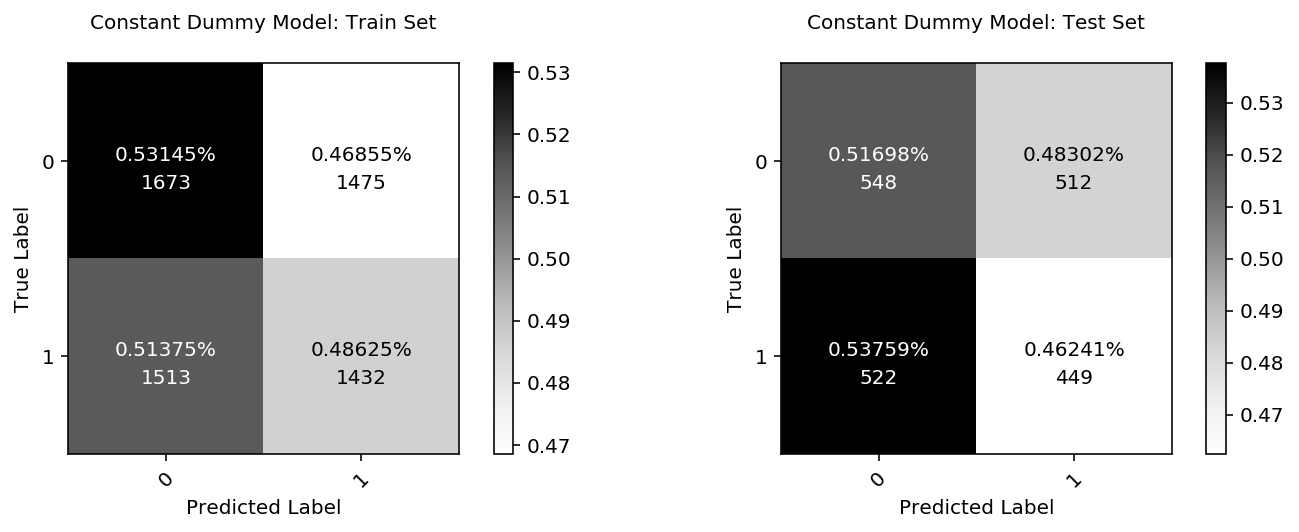### Stratified Dummy Model¶

A stratified dummy model looks at the overall distribution of classes in our target variable within our training set and then uses that information to assign a target value to each prediction randomly. Therefore in our case, it will notice that we have fewer poisonous mushrooms in our training set and adjust its prediction according. Again the results here are close to random.

In :
# Fit the intial logistic model
stratified_dm_model = DummyClassifier(strategy='stratified')
stratified_dm_model.fit(train_x, train_y);
scores = cross_val_score(stratified_dm_model, train_x, train_y, cv=10, scoring='accuracy')
print(f'Cross Validation Score: {scores.mean():.5f}')
plot_confusion_normal(stratified_dm_model, name ='Constant Dummy',
train_x=train_x, train_y=train_y, classes=[0,1],
test_x=test_x ,test_y=test_y, cmap=plt.cm.Greys)

Cross Validation Score: 0.50666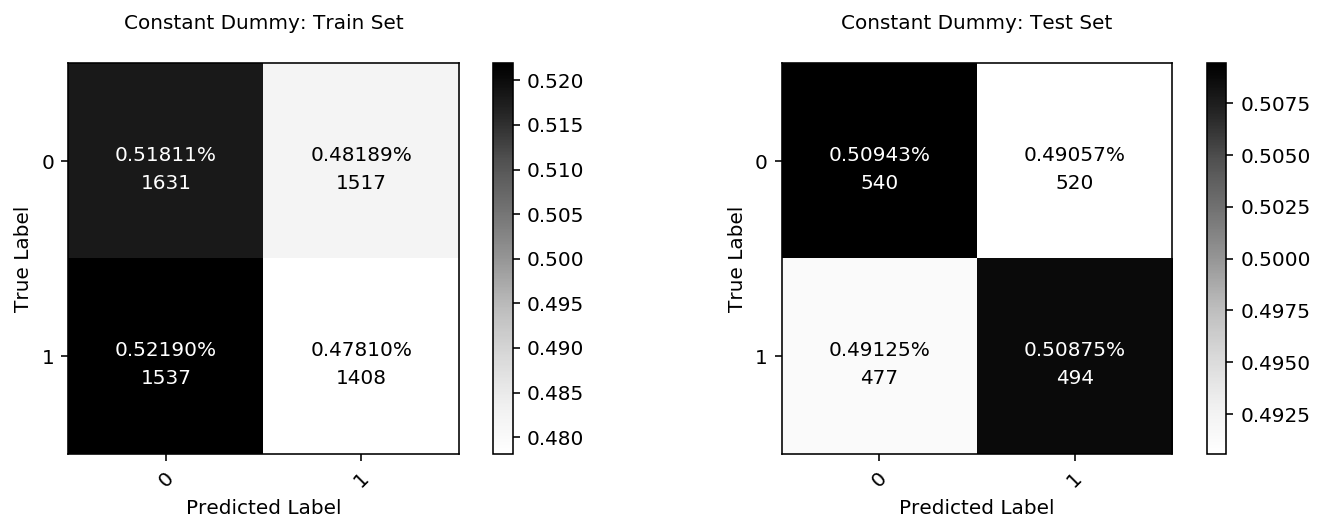### Uniform Dummy Model¶

The uniform strategy is the same as the stratified strategy, except it does not adjust for class imbalance. Therefore this dummy model predicts a class for every observation at random from the full range 'f target classes.

In :
# Fit the intial logistic model
random_dm_model = DummyClassifier(strategy='uniform')
random_dm_model.fit(train_x, train_y);
scores = cross_val_score(random_dm_model, train_x, train_y, cv=10, scoring='accuracy')
print(f'Cross Validation Score: {scores.mean():.5f}')
plot_confusion_normal(random_dm_model, name ='Uniform Dummy',
train_x=train_x, train_y=train_y, classes=[0,1],
test_x=test_x ,test_y=test_y, cmap=plt.cm.Greys)

Cross Validation Score: 0.50353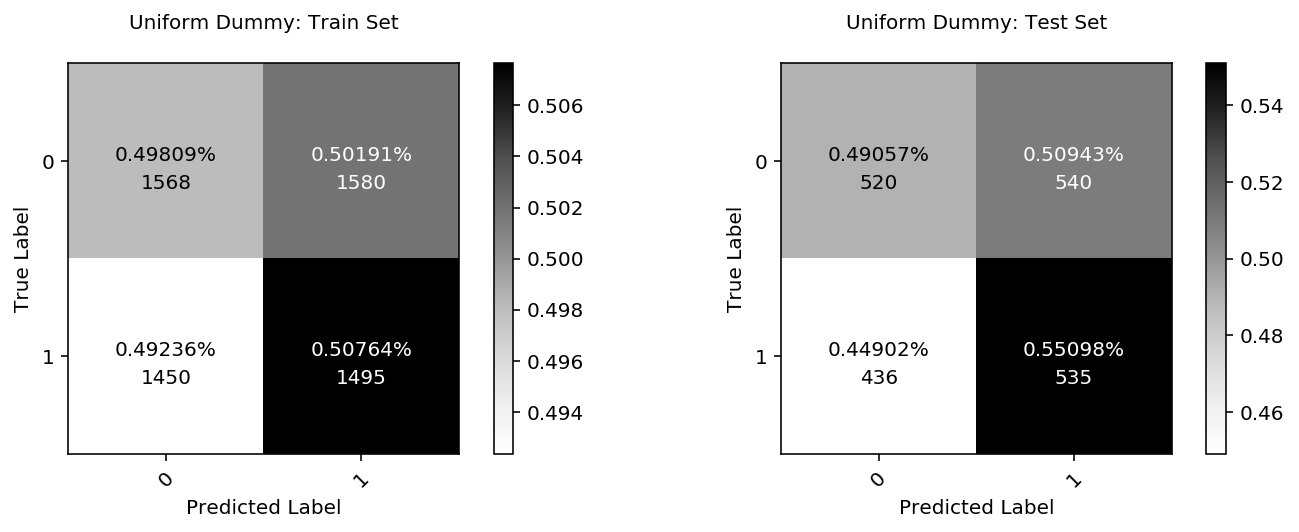### Prior Dummy Model¶

A prior strategy assumes that current observations must be related to the last training point, therefore assumes the target value of this observation is the True target value from the previous training point.

In :
# Fit the intial logistic model
prior_dm_model = DummyClassifier(strategy='prior')
prior_dm_model.fit(train_x, train_y);
scores = cross_val_score(prior_dm_model, train_x, train_y, cv=10, scoring='accuracy')
print(f'Cross Validation Score: {scores.mean():.5f}')
plot_confusion_normal(prior_dm_model, name ='Prior Dummy',
train_x=train_x, train_y=train_y, classes=[0,1],
test_x=test_x ,test_y=test_y, cmap=plt.cm.Greys)

Cross Validation Score: 0.51666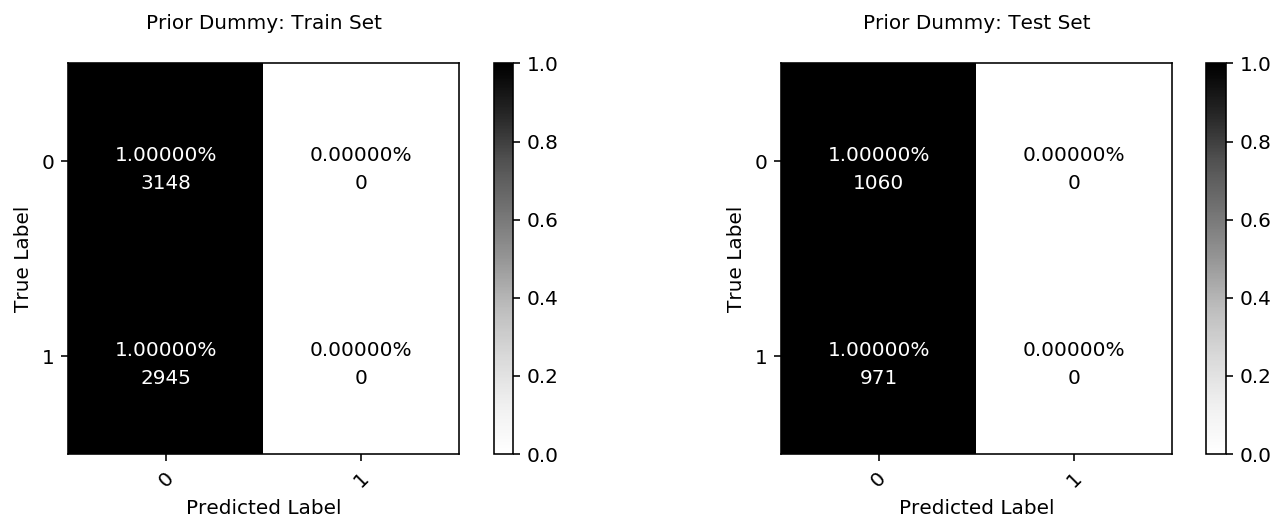### Logistic Regression¶

Depending on the problem, Any correctly trained model can beat the Sklearn dummy models. As you can see below, a simple logistic regression solves this problem very quickly.

In :
# Fit the intial logistic model
lg_model = LogisticRegression(C=10000)
lg_model.fit(train_x, train_y);
scores = cross_val_score(lg_model, train_x, train_y, cv=10, scoring='accuracy')
print(f'Cross Validation Score: {scores.mean():.5f}')
plot_confusion_normal(lg_model, name ='Linear Regression',
train_x=train_x, train_y=train_y, classes=[0,1],
test_x=test_x ,test_y=test_y, cmap=plt.cm.Blues)

Cross Validation Score: 1.00000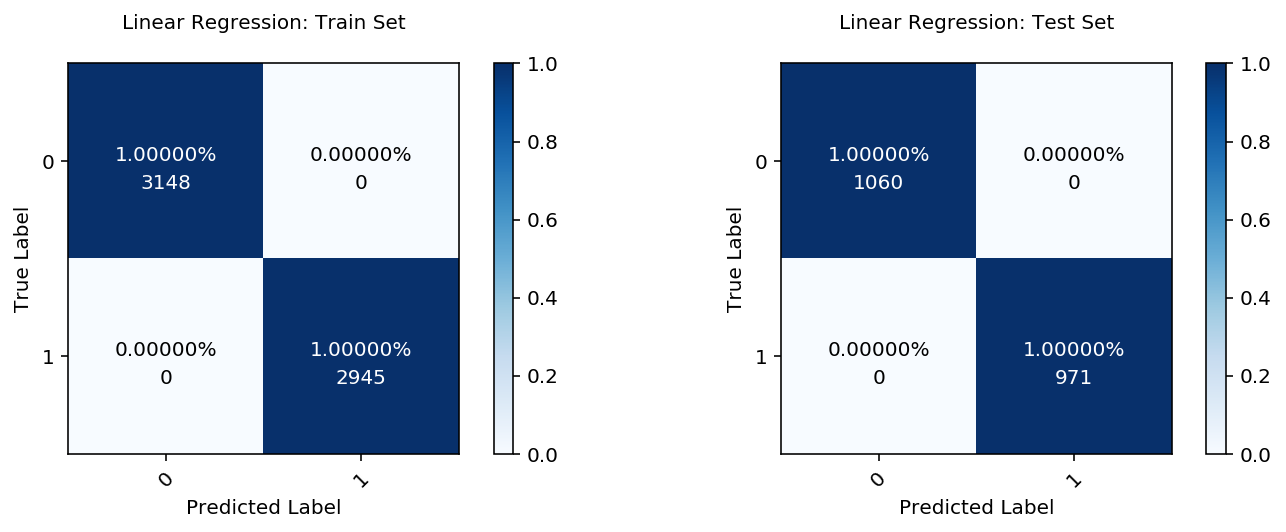Author: Kavi Sekhon